Tamilnadu State Board New Syllabus Samacheer Kalvi 9th Science Guide Pdf Chapter 3 Fluids Text Book Back Questions and Answers, Notes.

## Tamilnadu Samacheer Kalvi 9th Science Solutions Chapter 3 Fluids

### 9th Science Guide Fluids Text Book Back Questions and Answers

Question 1.
The size of an air bubble rising up in the water
(a) decreases
(b) increases
(c) remains the same
(d) may increase or decrease
(b) increasesQuestion 2.
Clouds float in the atmosphere because of their low
(a) density
(b) pressure
(c) velocity
(d) mass
(a) density

Question 3.
In a pressure cooker, the food is cooked faster because
(a) increased pressure lowers the boiling point.
(b) increased pressure raises the boiling point.
(c) decreased pressure raises the boiling point.
(d) increased pressure lowers the melting point.
(b) increased pressure raises the boiling point

Question 4.
An empty plastic bottle closed with an airtight stopper is pushed down into a bucket filled with water. As the bottle is pushed down, there is an increasing force on the bottom. This is because
(a) more volume of liquid is displaced.
(b) more weight of liquid is displaced.
(c) pressure increases with depth.
(d) All the above.
(c) pressure increases with depthII. Fill in the blanks :

1. The weight of the body immersed in a liquid appears to be ………………… than its actual weight
less

2. The instrument used to measure atmospheric pressure is …………
Barometer

3. The magnitude of buoyant force acting on an object immersed in a liquid depends on ……………. of the liquid.
density

4. A drinking straw works on the existence of ……………….
atmospheric pressureIII. State whether true or false. If false, correct the statement:

1. The weight of fluid displaced determines the buoyant force on an object.
True.

2. The shape of an object helps to determine whether the object will float or not.
False.
Correct statement: The density of an object helps to determine whether the object will floater sink.3. The foundations of high-rise buildings are kept wide so that they may exert more pressure on the ground.
False.
Correct statement: They may exert less pressure on the ground.

4. Archimedes’ principle can also be applied to gases.
True.

5. Hydraulic press is used in the extraction of oil from oilseeds.
True.

IV. Match the following :

 Density hpg 1 gwt Milk Pascal’s law Pressure Pressure exerted by a fluid Mass/Volume Lactometer 980 dyne

 Density Mass/Volume 1 gwt 980 dyne Pascal’s law Pressure Pressure exerted by a fluid hpg Lactometer Milk

Question 1.
On what factors the pressure exerted by the liquid depends on?
The pressure exerted by the liquid depends on the

• Depth
• Density of the liquid
• Acceleration due to gravity.

Question 2.
Why does a helium balloon float in the air?
Helium is much less dense than ordinary air which gives them buoyancy and thus floats in the air.Question 3.
Why it is easy to swim in river water than in seawater?
The question itself is wrong. It is easier to swim in seawater than in the river water. It is because seawater has
(i) greater density and
(ii) larger buoyant force than river water.

Question 4.
What is meant by atmospheric pressure?
The pressure exerted by the atmospheric gases on its surroundings and on the surface of the earth is called atmospheric pressure.

Question 5.
State Pascal’s law.
Pascal’s law: The external pressure applied to an incompressible liquid is transmitted uniformly throughout the liquid.Question 1.
An appropriate illustration proves that the force acting on a smaller area exerts a greater pressure.
Consider standing on loose sand. Your feet go deep into the sand. Now, when you lie down on the sand, you will find that your body will not go that deep into the sand. In both cases, the force exerted on the sand is the weight of your body which is the same. This force acting perpendicular to the surface is called thrust. When you stand on loose sand, the force is acting on an area equal to the area of your feet.

When you lie down, the same force acts on an area of your whole body, which is larger than the area of your feet. Therefore the effect of thrust, that is, the pressure depends on the area on which it acts. The effect of thrust on sand is larger while ‘ standing than lying.

Question 2.
Describe the construction and working of the mercury barometer.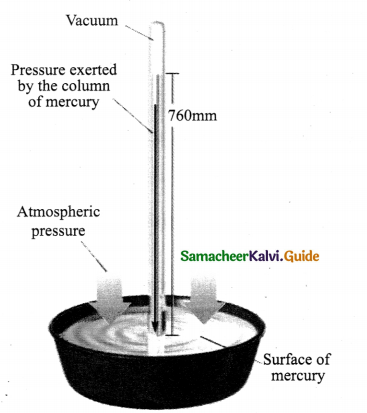Mercury Barometer
1. It is designed by Torricelli.

Construction :
2. Mercury Barometer consists of long glass tube closed at one end and opened at the other.
3. Mercury filled through open end and close that end by thumb and open it after immersing it into a trough of mercury.

Working:
4. The Barometer works by balancing the mercury in the glass tube against the outside air pressure.
5. If air pressure increases, it pushes more of the mercury up into the tub.
6. If air pressure decreases, more mercury drains from the tub.
7. As vacuum cannot exert pressure, Mercury in the tube provides a precise measure of air pressure which is called atmospheric pressure.
8. It is used in a laboratory or weather station.Question 3.
How does an object’s density determine whether the object will sink or float in water?
Whether an object will sink or float in a liquid is determined by the density of the object compared to the density of the liquid. If the density of a substance is less than the density of the liquid it will float. For example, a piece of wood which is less dense than water will float on it. Any substance having more density than water (for example, a stone), will sink into the water.

Question 4.
Explain the construction and working of a hydrometer with a diagram.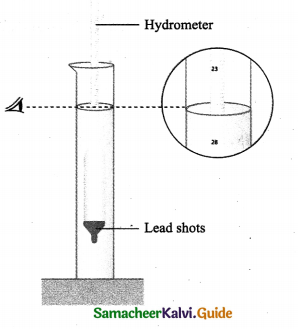Purpose:
To measure density (or) relative density of the liquid.Principle :
The weight of the liquid displaced by the immersed portion of the hydrometer is equal to the weight of the hydrometer. [Flotation principle].

Construction
Lower end of hydrometer :
A cylindrical stem having a spherical bulb which partially W Lead shots
filled with lead shots or mercury which helps to float or stand vertical in liquids.

Upper end of hydrometer:
A narrow tube has markings so that the relative density of liquids can be read off directly.

Working:

1. Liquid to be tested is poured into the glass jar.
2. The hydrometer is gently lowered into the liquid until it floats freely.
3. The reading against the level touching the tube gives the relative density of the liquid.

Question 5.
State the laws of flotation.
Laws of flotation are

• The weight of a floating body in a fluid is equal to the weight of the fluid displaced by the body.
• The centre of gravity of the floating body and the centre of buoyancy are in the same vertical line.VII. Assertion and Reason :

(a) If both assertion and reason are true and reason is the correct explanation of assertion.
(b) If both assertion and reason are true but reason is not the correct explanation of assertion.
(c ) If assertion is true but reason is false.
(d) If assertion is false but reason is true.

Question 1.
Assertion (A) : To float, body must displace liquid whose weight is equal to the actual weight.
Reason (R): The body will experience no net downward force in that case.
(a) Both assertion and reason are true and reason is the correct explanation of assertion]

Question 2.
Assertion (A) : Pascal’s law is the working principle of a hydraulic lift.
Reason (R): Pressure is thrust per unit area.
(b) Both assertion and reason are true but reason is not the correct explanation of assertion.]
Reason : Pascal’s law is the working principle of Hydraulic lift. In Hydraulic lift, applied pressure is transmitted uniformly and multiplied throughout the system.

VIII. Numerical Problems :

Question 1.
A block of wood of weight 200 g floats on the surface of water. If the volume of the block is 300 cm3, calculate the upthrust due to water.
Weight of woodblock, m = 200 g
Volume of the woodblock, V = 300cm3
Upthrust = Weight of the fluid displaced = Volume of the woodblock
Upthrust = 300 cm3Question 2.
Density of mercury is 13600 kg m-3. Calculate the relative density.
Density of Mercury = 13600 kg m-3
Density of water at 4°C= 1000 kg m-3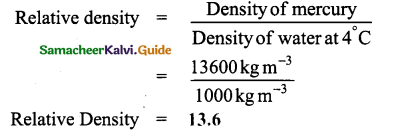Question 3.
The density of water is 1 g cm-3. What is its density in S.I. units?
Density of water in SI units = 1000 kg / m3

Question 4.
Calculate the apparent weight of wood floating on water if it weighs 100g in air.
Mass of wood = 100 g.
As the wood floats on the water, water will not be displaced.
So, the actual weight of wood is equal to the Apparent weight of wood.

IX. Higher Order Thinking Skills :

Question 1.
How high does the mercury barometer stand on a day when atmospheric pressure is 98.6 kPa?
Pressure of Atmosphere PatnT = 98.6 kPa.
Density of Mercury, ρHg = 13.6 × 103 kg/cm3
Acceleration due to gravity, g = 9.8 m/s2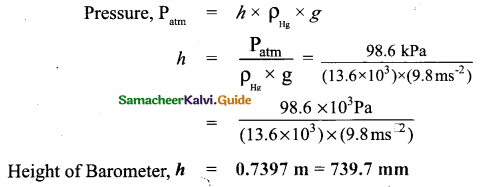Question 2.
How does a fish manage to rise up and move down in the water?

• Fish manages to rise up in the water by reducing its density by filling oxygen in the bladder via the gills. Thus volume will be increased to support its ascending motion.
• Fish moves down by decreasing its volume by releasing oxygen from the bladder. Thus volume will be decreased so it will sink in the water.

Question 3.
If you put one ice cube in a glass of water and another in a glass of alcohol, what would you observe? Explain your observations.
Ice cube in water: As the density of ice cube is less than water, the ice cube floats in water.
Ice cube in alcohol: As the density of the ice cube is greater than alcohol, the ice cube will sink in alcohol.
Note: Density : Water = 1.00, Ice cube = 0.917, Alcohol = 0.78

Question 4.
Why does a boat with a hole in the bottom would eventually sink?
A boat with a hole in the bottom eventually sinks due to :

• The water entered through a hole will increase the weight of the boat.
• The boat becomes heavier so it cannot displace more water. So the boat sinks.Intex activities

ACTIVITY – 1

Stand on the loose stand. Your feet go deep into the sand. Now, lie down on the sand. What happens? You will find that your body will not go that deep into the sand. Why?

Aim:
To demonstrate the effect of thrust

Materials Required:
Sand

Procedure:

1. First, you stand on the sand on your feet.
2. Lie down on the sand with your whole body.

Observation:

1. While standing on your feet on sand, your feet go deep into the sand.
2. While lying down with your body on the sand, your body will not go deep into the sand.

Conclusion:

1. Pressure depends upon the area on which it acts.
2. The effect of thrust on sand is larger while standing than lying.ACTIVITY – 2

Take a transparent plastic pipe. Also, take a balloon and tie it tightly over one end of the plastic pipe. Pour some water in the pipe from the top. What happens? The balloon tied at the bottom stretches and bulges out. It shows that the water poured in the pipe exerts pressure on the bottom of its container.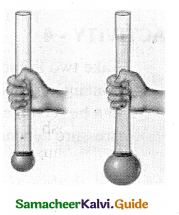Aim: To demonstrate that water exerts pressure on the bottom of the container.

Materials Required: Plastic pipe, Balloon, Water.

Procedure :

1. Take a transparent plastic pipe and a balloon.
2. Tie the balloon tightly over one end of the plastic pipe.
3. Keep the pipe with the closed end at the bottom.
4. Pour some water in the pipe from the top.

Observation: The balloon tied at the bottom stretches and bulges out.

Conclusion: Water poured in the pipe exerts pressure on the bottom of its container.

[End of the activity]ACTIVITY-3

Take a large plastic can. Punch holes with a nail in a vertical line on the side of the can as shown in the figure. Then fill the can with water. The water may just dribble out from the top hole, but with the increased speed at the bottom holes as depth causes the water to squirt out with more pressure.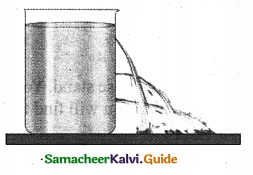Aim:
To demonstrate that pressure increases as depth increases.

Materials Required:

1. Large plastic can.
2. A sharp nail.

Procedure :

1. Take a large plastic can.
2. Punch holes with a nail in a vertical line up on the side of the can every inch or several centimeters.

Observation:

1. Water dribbles out from the top hole.
2. Water from the bottom hole flows with increased speed.

Conclusion:
Depth causes water to squirt out with more pressure.ACTIVITY – 4

Take two liquids of different densities say water and oil to the same level in two plastic containers. Make holes in the two containers at the same level. What do you see? It can be seen that water is squirting out with more pressure than oil. This indicates that pressure depends on density of the liquid.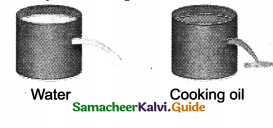Aim :
To demonstrate pressure depends on the density of the liquid.

Materials Required:

1. Two plastic containers
2. Water
3. Oil (Both same volume)
4. Sharp nail

Procedure:

1. Take water and oil to the same level in two plastic containers.
2. Make a hole at the same level in two containers.

Observation:
Water squirts out with more pressure than that of oil.

Conclusion:
Pressure depends on the density of the liquid.ACTIVITY – 5

Take two identical flasks and fill one flask with water to the 250 cm3 mark and the other with kerosene to the same 250 cm3 mark. Measure them in a balance. The flask filled with water will be heavier than the one filled with kerosene. Why? The answer is in finding the mass per unit volume of kerosene and water in respective flasks.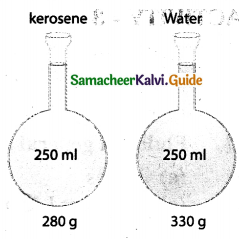Aim :
To prove that the density of a substance is the mass per unit volume of a given substance.

Materials Required:

2. Water
3. Kerosene (same volume as water)

Procedure :

2. Fill one flask with water to 250 cm3 mark.
3. Fill the other flask with kerosene to the same 250 cm3 mark.
4. Measure both flasks in balance separately.

Observation :
The flask filled with water will be heavier than that of the flask filled with kerosene.

Conclusion :

1. In the above activity, we know that Both water and kerosene have same volume (i.e.) 250 cm3.
2. The density of the water lg / cm3 and density of kerosene is 0.8g / cm3 mass
3. Density = $$\frac{\text { mass }}{\text { volume }}$$, therefore mass = Density x volume
Hence mass of water = 1g/cm3 x 250 cm3 = 250g
mass of kerosene = 0.8 g / cm3 x 250 cm3 = 200g
4. Even though, water and kerosene have same volume, they have different densities. So water and kerosene have different masses.
5. Water has more mass than kerosene.
Hence, we proved that the density of the substance is the mass per unit volume of the substance. [End of the activity]I. Choose the correct answer :

Question 1.
Intermolecular forces are stronger in ………………
(a) gases
(b) liquids
(c) solids
(d) all the above
(c) solidsQuestion 2.
Water (or) liquids exert pressure on
(a) Upward direction
(b) Downward direction
(c) Lateral direction
(d) All the above
(d) All the above

Question 3.
The pressure does not depend upon
(a) Depth
(b) Area
(c) Density
(d) Acceleration due to gravity
(b) Area

Question 4.
Fluids in general are
(a) Gases
(b) liquids
(c) Gases or Liquids
(d) None of these
(c) Gases or LiquidsQuestion 5.
Scuba divers wear special suits to withstand
(a) Low pressure
(b) High pressure
(c) Low temperature
(d) High temperature
(b) High pressure

Question 6.
To find out relative density of the substance, with respect to density of water……………C is taken.
(a) 4°
(b) 0°
(c) 100°
(d) 60°
(a) 4°

Question 7.
Density Bottle is also called as
(a) Saccharometer
(b) Lactometer
(c) Pycnometer
(d) Barometer
(c) PycnometerQuestion 8.
An object completely immersed in fluid displaces its own volume of fluid.
(a) Floatation principle
(b) Principle of buoyancy
(c) Pascal’s law
(d) Archimedes principle
(d) Archimedes principle

Question 9.
A solid floats in liquid with a portion of it being submerged. Then
(a) The liquid exerts an upthrust equal to weight of the solid
(b) The weight of the dispersed liquid is equal to the weight of solid
(c) Solid exerts a force equal to its weight on liquid
Choose correct statements
(A) a & b
(B) a & c
(C) b & c
(D) All of these
(A) a & b

Question 10.
The principle of “Hydrostatic balance” was devised by
(a) Torricelli
(b) Pascal
(c) Archimedes
(d) Newton
(c) ArchimedesQuestion 11.
Saccharometer is used to measure the density of …………….in a liquid.
(a) Milk
(b) Sugar
(c) Alcohol
(d) Ether
(b) Sugar

Question 12.
Most buoyant objects are those with relatively
(a) high volume
(b) higher mass
(c) low density
(d) less viscosity
(A) a & b (B) a & c (C) b & c (D) b&d
(B) a & c

Question 13.
If there were no gravity, which of the following will not be there for fluid? (HOTS)
(a) Viscosity
(b) Density
(c) Pressure
(d) upthrust
(d) upthrustQuestion 14.
Human lung is well adopted to breath at a pressure of ………….kPa.
(a) 106.7
(b) 101.3
(c) 98.4
(d) 33.7
(b) 101.3

Question 15.
Petroleum-based products float on the surface of the water. This is due to their low ………….
(a) volume
(b) density
(c) specific gravity
(d) viscosity
(A) a & b (B) a & c (C) a & d (D) b & c
(D) b & cII. Fill in the blanks :

1. It is easy to compress a gas whereas liquids are ………………….
Incompressible

2. The net force in a particular direction is called ………………….
Thrust

3. All flowing substances, both liquids, and gases are called ………………….
Fluids4. The air pressure at sea level is referred as ………………….
Atmospheric pressure

5. The pressure in mines is ………………….than sea level.
Greater

6. ………………….is the instrument used to measure the atmospheric pressure.
Barometer

7. On each lm2 of surface, the force acting is ………………….
1.013 kN

8. ………………….is a device for measuring atmospheric pressure without the use of liquids.
Aneroid Barometer9. Absolute Pressure is zero-referenced against a ………………….
Perfect Vaccum

10. Psi stands for ………………….
Pascal per inch

11. A tyre pressure of 30psi is almost ………………….the atmospheric pressure.
Twice

12. The density of the substance is the …………………. of a given substance.
mass per unit volume

13. Hydrometer is based on the principle of ………………….
Flotation

14. The upward force that is caused due to the pressure difference in liquid (or fluid) is called ………………….
Buoyant force15. Hot air is ………………….dense than ordinary air.
less

16. The Lactometer works on the principle of ………………….of milk.
gravity

17. Icebergs and ships stay afloat due to ………………….
Buoyancy

18. Archimedes principle is the consequence of ………………….
Pascal’s law

19. The point in which the force of buoyancy is supposed to act is known as …………………..
Centre of buoyancy

20. The centre of gravity of the floating body and the centre of buoyance are in the same ………………….line.
Vertical21. In a fluid, buoyant force exists because the pressure at the ………………….of an object is greater than the pressure at the top.
bottom

III. Match the following :

(I)

 1. Lactometer a) Relative density 2. Saccharometer b) Alcohol 3. Alcoholometer c) Sugar 4. Pyncometer d) Milk

1. d
2. c
3. b
4. a(II)

 1.Hydraulic press a) Archimedes 2.Cartesian Diver b) Floatation 3. Hydrostatic Balance c) Pascal’s law 4. Hydrometer d) Buoyancy

1. c
2. d
3. a
4. b

IV. State whether true or false. If false, correct the statement:

1. The shape and size of the solids do not easily change.
True.

2. Liquid exerts pressure in the upward direction.
False.
Correct statement: Liquid exerts pressure in all directions.3. The barometer works by balancing the Mercury in the glass tube along with the outside air pressure.
False.
Correct statement: The barometer works by balancing the Mercury in the glass tube against the outside air pressure.

4. The absolute pressure is zero-referenced against atmospheric pressure.
False.

Correct statement: The absolute pressure is zero-referenced against a perfect vacuum.

5. The external pressure applied on an incompressible liquid is transmitted uniformly throughout the liquid.
True.

6. The correct lactometer reading is only obtained at a temperature of 60° C.
True.7. If the buoyant force is less, the object will float.
False.
Correct statement: If the buoyant force is less, the object will sink.

8. If the volume of object is above the water surface, then the object is less densed.
True.

9. Upthrust = weight of the fluid displaced – apparent weight of the object.
False.
Correct statement: Upthrust = Weight of the fluid displaced – apparent loss of weight of the object.

10. Salt water provides less buoyant force than freshwater.
False.
Correct statement: Salt water provides more buoyant force than freshwater.V. Very Short Answer Questions :

Question 1.
Differentiate Liquid from Gas.
It is easy to compress a Gas. The liquid is Incompressible.

Question 2.
What is the SI unit of pressure?
Newton per squaremeter (Nm-2).

Question 3.
What are factors determining liquid pressure?
(i) Depth (b) (ii) Density bf Liquid (□) (iii) Acceleration due to gravity (g).

Question 4.
Write the equation for pressure due to liquid column.
P = hg ; P – Pressure, h- depth, p- density, g – Acceleration due to gravity.Question 5.
What is referred to as atmospheric pressure?
Air pressure at sea level is referred to as atmospheric pressure.

Question 6.
Expand the abbreviation ‘psi’.
Psi = Pascal per inch.

Question 7.
What are Force multipliers?
Hydraulic systems are known as-force multipliers.

Question 8.
Write the SI unit & symbol for density?
SI unit = kilogram per meter cube (kg / m3).
Symbol = rho (ρ).Question 9.
Where do we use lactometers?
In milk processing units and Dairies.

Question 1.
What happens when pressure is increased in solids?
If the pressure is increased in solids

• it experiences tension
• it ultimately deforms (or) breaks.Question 2.
How will you calculate fluid pressure?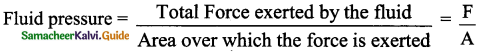Question 3.
How will you find the absolute pressure?

1. For pressures higher than atmospheric pressure:
Absolute pressure = Atmospheric pressure + Gauge pressure.
2. For pressures lower than atmospheric pressure:
Absolute pressure = Atmospheric pressure – Gauge pressure.Question 4.
Why do Scuba divers wear special suits and equipment?

1. Deep-sea has pressure twice that of atmospheric pressure.
2. At high pressure, parts of our body including blood vessels & soft tissues cannot withstand it.
Hence they use special suits & equipment for protection.

Question 5.
Define Relative Density.
Relative density of a substance is defined as ratio of density of substance to density of water at 4°C.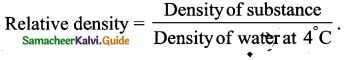Question 6.
Name different types of Hydrometers with their applications.

 Name of Hydrometer Application (measuring) 1. Lactometer Density of milk 2. Saccharometer Density of sugar in a liquid 3. Alcoholometer Higher levels of alcohols in Spirits

Question 7.
What do you understand by the term “Buoyancy”.
When a body partially or completely immersed in a liquid (fluid), the pressure is more at the bottom and less at the surface in the liquid.
This Pressure difference causes an upward force called “Buoyant force”. The phenomenon is called ‘Buoyancy’.Question 8.
How do submarines sink and float in water?
Submarines change the level of floating by pumping in and pumping out water into its compartments.

Question 9.
Differentiate positive & negative buoyant.
Positive Buoyant

1. Weight of the object is less than the amount of water displaced.
2. More buoyant force
3. Object will float

Negative Buoyant :

1. Weight of the object is more than the amount of water displaced.
2. Less Buoyant force.
3. Object will sink.Question 10.
You have a bag of cotton and an iron bar, each indicating a mass of 100 kg when measured on a weighing machine. In reality, one is heavier than the other. Can you say which one is heavier and why?
The bag containing the iron bar is heavier than cotton.
Reason: Although both of them have the same weight, the bag of the iron bar has less volume so more dense compared to the bag of cotton which has more volume and less dense.

Question 1.
Derive an expression for Pressure due to the Liquid column.
A tall beaker filled with water to form a liquid column
Area of the cross-section at bottom = A
Height of liquid column = h
The density of the liquid = ρ
Thrust at bottom of liquid column (F) = Weight of liquid.
F = mg …(1) (∵ m – mass of liquid)
Mass,m = ρ × V ……………… (2)
Volume of liquid columñ, V = Area of cross-section (A) × height (h)
V = Ah ………….(3)
Substitute (3) in (2) n, = ρ Ah ………………… (4)
Substitute (4) in (1) F = ρ Ahg ……………….(5)
Pressure (P) = $$\frac{\text { Thrust (F) }}{\text { Area (A) }}=\frac{\rho \text { A h g }}{\mathrm{A}}$$
∴ P = hpg – This is the expression for pressure due to the liquid column.Question 2.
Describe the construction and working of the Pycnometer.
Pycnometer (Density Bottle)

Purpose: To measure relative density.

Construction :

1. Pycnometer consists of a ground glass stopper with a fine hole through it.
2. When the bottle is filled and the stopper is inserted, the excess liquid rises through the hole and runs down outside the bottle.

Working:

1. The bottle will always contain the same volume of liquid at a constant temperature.
2. The density of the given volume of substance to the density of equal volume of referred substance is called relative density or specific gravity of the substance.

Question 3.
Explain the Archimedes principle with an example.
Principle:
A body immersed in a fluid experiences a vertical upward buoyant force equal to the fluid it displaces.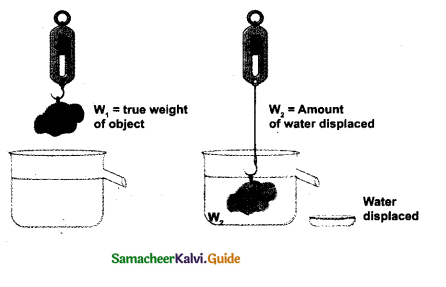Explanation:
(1) When a body is partially or completely immersed in a fluid at rest, it experiences an upthrust which is equal to the weight of the fluid displaced by it.

(2) Due to the upthrust, the body loses a part of its weight equal to upthrust.
Upthrust = Weight of the fluid displaced.
= Apparent loss of weight of the body.
Apparent weight of an object = True weight of object in air – upthrust.

Question 4.
Describe the purpose, principle and working of Lactometer.
Purpose: Lactometer is an instrument to check the purity of milk.
Principle: Gravity of milk.

Construction :

1. Lactometer consists of a long graduated test tube with a cylindrical bulb.
2. The cylindrical bulb has graduation from 15 at the top and 45 at the bottom, which filled with mercury.
3. The test tube is filled with water.
4. The air chamber causes the instrument to float.
5. Mercury causes lactometer to sink up proper level and to float in an upright position in the milk.
6. There is a thermometer inside the lactometer that extends to the upper part of test tube.

Working:

1. The correct lactometer reading is only obtained at 60°C.
2. Lactometer measures the cream (density) content of milk.
3. Lactometer floats in milk if milk has more cream content.
4. The average reading of normal milk is 32.VIII. Numerical Problems :

Question 1.
A vessel with water is placed on a weighing pan and it reads 600 g. Now a ball of mass 40 g and density is 0.80g / cm3 is sunk into the water with a pin of negligible volume as shown in the figure. The weighing pan will show the reading of …………….?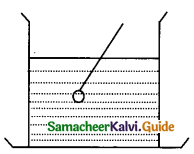Solution :
Weight of vessel with water = 600g
Mass of ball = 40g
Density of bal = 0.80 g / cm3
Volume of the ball = $$\frac{\text { mass }}{\text { density }}=\frac{40}{0.80}$$= 50g
So, weight of vessel + volume of ball = 600 + 50 g
The weighing pan will show = 650g
The weighing pan will show = 650g
The reading of a spring balance when a block is suspended from it in air is 60 newton. This reading is changed to 40 newton when the block is submerged in water. Calculate the specific gravity of block.
60 – 40 = 20 newton Weight of block in air
Loss of weight in water 60-newton / 20 newton = 3Question 2.
The reading of a spring balance when a block is suspended from it in air is 60 newton. This reading is changed to 40 newtons when the block ¡s submerged in water. Calculate the specific gravity of block.
Solution:
Weight of block in air = 60 newton
Loss of weight of block in water = 60 – 40 = 20 newton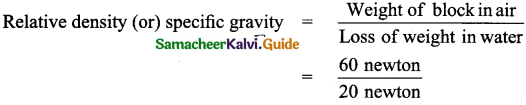The specific gravity of block = 3

Question 3.
The mass of a body ¡s 4 kg and its volume is 500 cm3. Find its relative density.
Solution:
Massofthebodym = 4kg = 4000g
Volume ofthebodyv = 500 cm3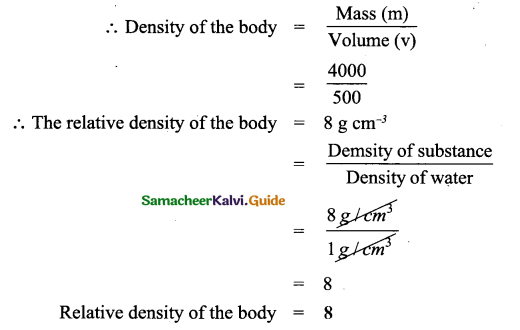Question 4.
Calculate the pressure produced by a force of 800 N acting on an area of 2.0 m2
Solution :
Force = 800 N
Area = 2.0m2
Pressure, P =$$\frac{\text { Force }}{\text { Area }}=\frac{800}{2.0}$$ = 400 Nm-2
Pressure P = 400 Nm-2 (or) 400 Pa

Question 5.
A swimming pool of width 9.0 m and length 24.0 m is filled with water of depth 3.0 m. Calculate the pressure on the bottom of the pool due to the water.
Solution:
Width of the pool, b = 9.0 m
Length of the pool, h = 24.0 m
Depth ofthepool,h = 3.0m
Density of water, p 1000 kg/m3
Pressure due to column of Fluid, P = ρhg
Acceleration due to gravity, g = 9.8 m/s2
Substituting the values, P = ρhg
P = 100kgm-3 × (3.0m) × (9.8ms-2)
Pressure, P = 29400kgm-1s-2      ∵1Pa = 1kgm-1s-2
∴P = 29400 Nm-2 (or) 29400PaQuestion 6.
A body of volume 100 cc is immersed completely in the weight of water and the jar before immersion of the weight of water and jar after immersion.
Volume of body completely immersed in water, V = = 100cc
Weight of water and jar before Immersion = 700g
The volume of jar immersed in water = Volume of water displaced = 100cc
Density of water = 1g/cm3
Mass of water displaced = Apparent weight loss
Mass of water displaced = Volume × density
= 100cc × 1g/cm3
Apparent weight loss of body = 100 g
Weight of jar and water after immersion = Weight of water and jar before immersion – Apparent weight loss
= 700g – 100g
= 600g.

IX. Assertion and Reason :

(a) Mark the correct choice as:
(a) If both Assertion and Reason are true and Reason is the correct explanation of Assertion.
(b) If both assertion and reason are true but reason is not the correct explanation of assertion.
(c) If assertion is true but reason is false.
(d) If assertion is false but reason is true.

Question 1.
Assertion (A) : The buoyant force on submerged rigid object can be considered to be acting at the centre of mass of object.
Reason (R) : In rigid body, force distributed uniformly through its volume can be considered to be acting at the centre of mass of the body.
(c) Assertion is true but reason is false]
Reason : Centre of the mass of the body is fixed according to the distribution of density.

Question 2.
Assertion (A): The weight of the truck exerts less pressure on road.
Reason (R): The truck has six to eight wheels. As area increases pressure decreases.
(a) Both Assertion and Reason are true and Reason is the correct explanation of Assertion

Question 3.
Assertion (A): Air gets thinner with increasing altitude.
Reason (R): The atmospheric pressure increases as we go up in mountains.
(c) Assertion is true but the reason is false
Reason: The atmospheric pressure decreases as we go up in mountains.

Question 4.
Assertion (A) : Lactometer is used to check the purity of milk.
Reason (R) : Lactometer measures the cream content of milk.
(b) Both assertion and reason are true but the reason is not the correct explanation of the assertion
(b) Directions: In each of the following questions, a statement of Assertion (A) is given followed by a corresponding statement of Reason (R) just below it. Of the statements, mark the correct answer as :Question 5.
Assertion (A) : The force acting on the surface of a liquid at rest, under gravity, in a container is always horizontal.
Reason (R) : The forces acting on a fluid at rest have to be normal to the surface.
(d) Assertion is false but reason is true
Reason: The force acting on the surface of liquid at rest, under gravity, in a container is always perpendicular due to the fact that molecules at the surface is attracted by the molecules below the surface (i.e) an inward attraction.

Question 6.
Assertion (A): A sleeping mattress is so designed that when you lie on it, a large area of your body comes in its contact.
Reason (R) : This reduces the pressure on the body and sleeping becomes comfortable.
(a) Both Assertion and Reason are true and Reason is the correct explanation of Assertion]

Question 7.
Assertion (A): Wide wooden sleepers are kept below railway lines to reduce pressure on the railway tracks and prevent them from sinking in the ground.
Reason (R): Pressure is directly proportional to the area in which it is acting.
(c) Assertion is true but the reason is false
Reason: Pressure is inversely proportional to the area in which it is acting.X. Define the following

1. Define thrust: The force which produces compression is called thrust. Its S.I units is newton
2. Define pressure: Thrust acting normally to a unit area of a surface is called pressure. Its S.I. Unit is the pascal.
3. Define atmospheric pressure: The pressure exerted by the atmospheric gases on its surroundings and on the surface of the earth is called atmospheric pressure. 1 atm is the pressure exerted by a vertical column of mercury of 76 cm height.
4. Buoyant force: The upward force experienced by a body when partly or fully immersed in a fluid is called upthrust or buoyant force.
5. Pascal’s law: Pascal’s law states that an increase in pressure at any point inside a liquid at rest is transmitted equally and without any change, in all directions to every other point in the liquid.
6. Archimedes principle: Archimedes’ principle states that when a body is partially or wholly immersed in a fluid, it experiences an up thrust or apparent loss of weight, which is equal to the weight of the fluid displaced by the immersed part of the body.
7. Density: Density is known as mass per unit volume of a body. Its S.I. unit is kg nr5.
8. Relative density: Relative density is the ratio between the density of a substance and the density of water. The relative density of a body is a pure number and has no unit.
9. Hydrometer: A hydrometer is a device used to measure the relative density of liquids based on Archimedes’ principle.
10. Lactometer: Lactometer is a device used to check the purity of milk by measuring its density using Archimedes’principle.